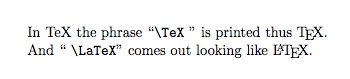try.html for testing
21may10

\begin{document}
\maketitleThis is an experiment with verbatim

$\sqrt{2}$

%place text here
In TeX the phrase \verb=\TeX= " is printed thus \TeX. \\
And  \verb=\LaTeX=" comes out looking like \LaTeX.

Rats, ltmml.js does not have the \verb function built in, but
something happens, it shaves off the space followed by the f
in function.

Note that in HTML the < and > are inescapable unless their
content is not recognizable (if incomprehensible then print it
literally, just as in TeX, "backslash unknown" prints that.
Hence, we trick HTML by using the "ampersand identifier semicolon"
syntax as below.

< div class="literalblock" >
< div class="content" >
< pre >
< tt >
e^&#960;i^+1 = 0. H~2~O and x^10^. Some ^super text^
and \~some sub text\~ except that the tildes around "some sub text"
don't make it if the div is still inside lttml because of the LaTeX
space meaning. On the other hand, escaping the tilde
</tt>
</pre>
< /div>
< /div>

I think that mixing HTML with TeX is not good for writing about
either languange, but is good for making web readable geometrical
documents.

\end{document}